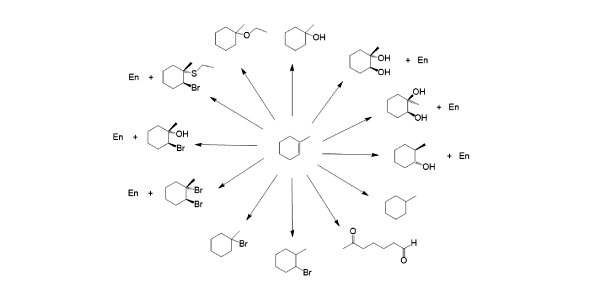# Alkene Reactions Trivia Quiz!

15 Questions | Total Attempts: 1671SettingsDo you know anything about Alkene's reactions? Would you like to try this quiz? Alkenes are primarily stable compounds but are more reactive than alkenes, either because of the carbon-carbon pi-bond's reactivity or the existence of allylic CH centers. Most reactions of alkenes involve accumulations to this pi bond, creating new single bonds. If you need to know about alkene reactions for an upcoming exam, or if you are simply curious,see if you and this quiz have chemistry.

• 1.
Addition of HCl, HBr, HI to an alkene is:
• A.

Markovnikov

• B.

Non-Markovnikov

• C.

Syn

• D.

Anti

• E.

Yields a ring

• 2.
The addition of Cl2 and Br2 to an alkene is:
• A.

Markovnikov

• B.

Non-Markovnikov

• C.

Syn

• D.

Anti

• E.

Yields a ring

• 3.
The addition of X2 and H2O to an alkene is:
• A.

Markovnikov

• B.

Non-Markovnikov

• C.

Syn

• D.

Anti

• E.

Yields a ring

• 4.
Reaction of Hg(OAc)2, H2O/THF, NaBH4 with an alkene is:
• A.

Markovnikov

• B.

Non-Markovnikov

• C.

Syn

• D.

Anti

• E.

Yields a ring

• 5.
Reaction of BH3, THF, H2O2, OH- with alkene is:
• A.

Markovnikov

• B.

Non-Markovnikov

• C.

Syn

• D.

Anti

• E.

Yields a ring

• 6.
Reaction of alkene with CHCl3 and KOH is:
• A.

Markovnikov

• B.

Non-Markovnikov

• C.

Syn

• D.

Anti

• E.

Yields a ring

• 7.
Reaction of alkene with CH2I2 and Zn(Cu) and ether is:
• A.

Markovnikov

• B.

Non-Markovnikov

• C.

Syn

• D.

Anti

• E.

Yields a ring

• 8.
Reaction of alkene with H2 and Pd/C or PtO2 is:
• A.

Markovnikov

• B.

Non-Markovnikov

• C.

Syn

• D.

Anti

• E.

Yields a ring

• 9.
Reaction of alkene with a peroxyacid (RCOOOH) is:
• A.

Markovnikov

• B.

Non-Markovnikov

• C.

Syn

• D.

Anti

• E.

Yields a ring

• 10.
Reaction of an epoxide (has a ring of two carbons and an oxygen) with H3O+ is:
• A.

Markovnikov

• B.

Non-Markovnikov

• C.

Syn

• D.

Anti

• E.

Yields a ring

• 11.
Reaction of alkene with OsO4, NaHSO3, H2O or with OsO4, NMO is:
• A.

Markovnikov

• B.

Non-Markovnikov

• C.

Syn

• D.

Anti

• E.

Yields a ring

• 12.
Reaction of alkene with O3 and Zn/H3O+ is:
• A.

• B.

Cleavage

• C.

Substitution

• 13.
Reaction of alkene with KMnO4 in H3O+ is:
• A.

• B.

Cleavage

• C.

Substitution

• 14.
Reaction of a diol with HIO4, H2O is:
• A.

• B.

Cleavage

• C.

Substitution

• 15.
Addition of alkene and aqueous acid is:
• A.

Markovnikov

• B.

Non-Markovnikov

Related TopicsBack to top